SPSS TUTORIALS BASICS ANOVA REGRESSION FACTOR CORRELATION

# SPSS with Python – Looping over Scatterplots

The right way for looping over tables, charts and other procedures in SPSS is with Python. We'll show how to do so on some real world examples. We'll use alcotest.sav throughout, part of which is shown below.
Note that you need to have the SPSS Python Essentials properly installed for running these examples on your own computer.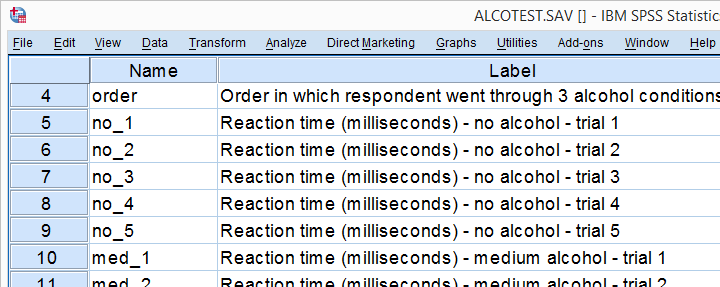## Example 1: Simple Loop over Bar Charts

We'd like to visualize how mean reaction times are related to the order in which people went through the 3 alcohol conditions. We'll start by generating the syntax for the first chart from the menu as shown below.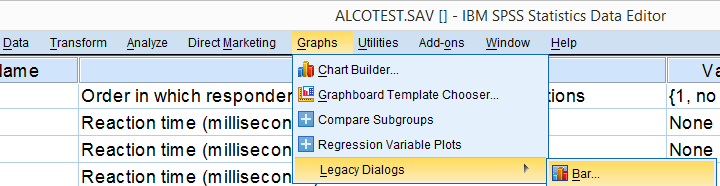As a rule of thumb, try to use Legacy Dialogs for generating charts. The interface and resulting syntax are wonderfully simple and often result in the exact same charts as the much more complex Chart Builder.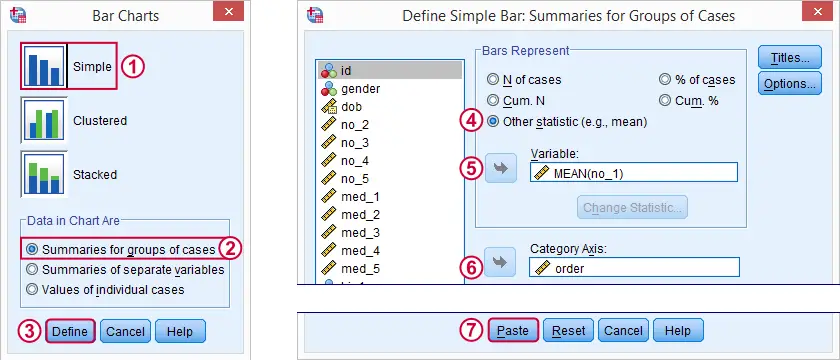We'll remove all line breaks from the pasted syntax, resulting in GRAPH /BAR(SIMPLE)=MEAN(no_1) BY order. Running this line results the first desired bar chart. For running similar charts over different reaction times, we could copy-paste the line and replace `no_1` by `no_2` and so on. However, a cleaner way to go is with the Python syntax below.

## SPSS Python Loop Syntax 1

*Specify variable names manually as Python list object and just print it.

begin program.
import spss
varList = ['no_1','no_2','no_3','no_4','no_5']
print varList
end program.

*If variable list ok, loop over it.

begin program.
for var in varList:
spss.Submit('''
GRAPH /BAR(SIMPLE)=MEAN(%s) BY order.
'''%(var))
end program.

## Note

You'll probably recognize the bar chart syntax near the end of the second block. The only difference is that the variable name has been replaced by `%s`. This is a Python string placeholder and it'll be replaced by a different variable name in each iteration.

## Result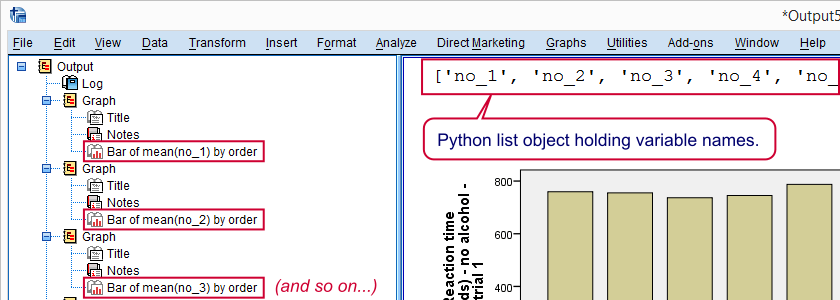## Example 2: Look Up Variable Names from Data

One thing we don't like about the first example is spelling out the variable names. Python can retrieve them from your data in many ways. An approach that always works is specifying variable names with the SPSS TO and ALL keywords. As shown below, the specification can be expanded into a Python list over which you can loop as desired.

*Retrieve variable names from data and print for inspection.

begin program.
import spss,spssaux
varSpec = "no_1 to hi_5" #Specify variables with SPSS TO or ALL keywords
varDict = spssaux.VariableDict(caseless = True)
varList = varDict.expand(varSpec)
varList.sort(key = lambda x: varDict.VariableIndex(x))
print varList
end program.

*If variable list ok, loop over it.

begin program.
for var in varList:
spss.Submit('''
GRAPH /BAR(SIMPLE)=MEAN(%s) BY order.
'''%(var))
end program.

## Example 3: Parallel Looping

We'd now like to inspect scatterplots of reaction times of no alcohol versus medium alcohol over each of the 5 trials. Like previously, we'll first generate syntax for just one scatterplot as shown below.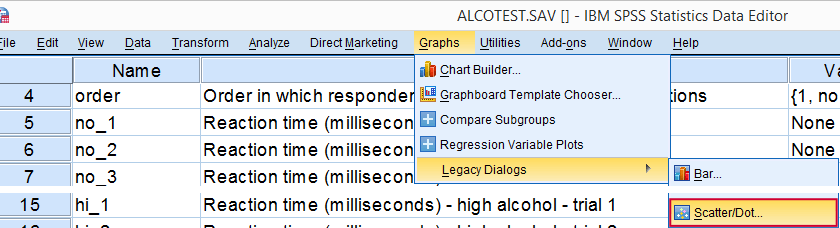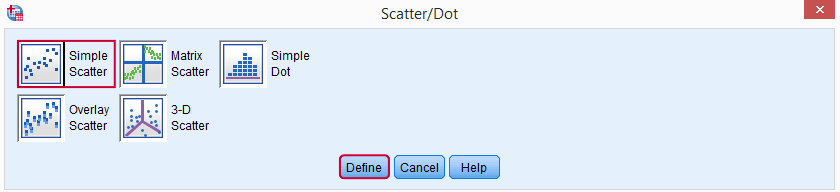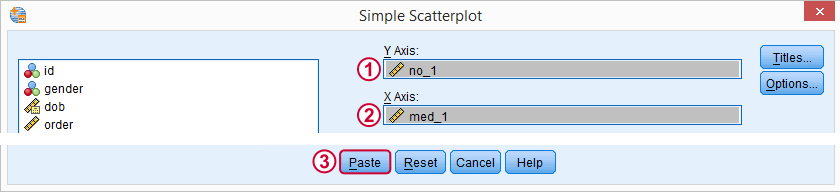After removing all line breaks, these steps result in GRAPH /SCATTERPLOT(BIVAR)=med_1 WITH no_1 /MISSING=LISTWISE.

## Retrieving Variable Names by Pattern

The syntax below sets up two empty Python lists and loops over all variable names in our data. Variable names starting with “no_” are added to one list and those that start with “med_” go into the other. Finally, we'll loop over both lists in parallel for generating our scatterplots.

*Retrieve variable names by pattern in name and print them.

begin program.
import spss
noVars,medVars = [],[] #set up two empty lists
for varInd in range(spss.GetVariableCount()): #loop over all variable indices
varName = spss.GetVariableName(varInd)
if varName.startswith('no_'): #if pattern in variable name...
elif varName.startswith('med_'):
medVars.append(varName)
print noVars,medVars
end program.

*If variable lists ok, run parallel loop over them.

begin program.
for listInd in range(len(noVars)):
spss.Submit('''
GRAPH /SCATTERPLOT(BIVAR)= %s WITH %s /MISSING=LISTWISE.
'''%(noVars[listInd],medVars[listInd]))
end program.

## Note

The second block loops over list indices (“listInd”) that refer to the first, second, ... element in either list. Python then retrieves the first, second, ... variable name from either list with `noVars[listInd]`.

## Example 4: Create Variable Names with Concatenation

We'll now show an easier option for our scatterplots that'll work if variable names end in simple numeric suffixes. We'll simply loop over a list holding numbers 1 through 5 (generated by `range(1,6)`) and concatenate these numbers to the variable name roots.

*Generate variable names by concatenating variable name root with numeric suffix.

begin program.
import spss
for varSuffix in range(1,6): #range(1,6) evaluates to [1, 2, 3, 4, 5]
spss.Submit('''
GRAPH /SCATTERPLOT(BIVAR)=no_%(varSuffix)d WITH med_%(varSuffix)d /MISSING=LISTWISE.
'''%locals())
end program.

## Note

In Python, `%d` is a general integer placeholder. It's replaced by some integer number that's specified later.
Alternatively, `%(varSuffix)d` is replaced by the integer number in `varSuffix` if `%locals()` is specified at the end. Using `%locals()` makes your code more readable and shorter, especially with multiple (text or number) placeholders.

## Example 5: Lower Triangular Loop

Our final example creates all possible different scatterplots among a set of variables. That is, if we'd run a correlation matrix of these variables, each cell underneath the main diagonal (hence “lower triangle”) is visualized in a scatterplot. This time we'll look up the variable names by their indices under variable view as shown below.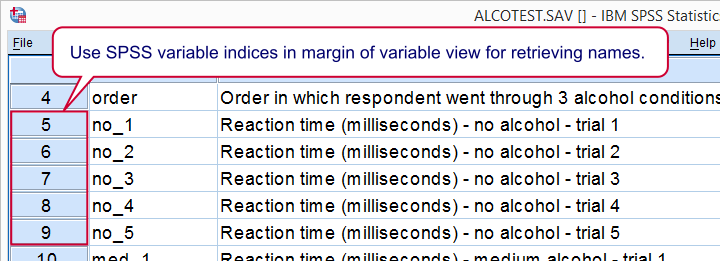## Syntax

*Retrieve variable names by indices.

begin program.
import spss,spssaux
noVars = spssaux.GetVariableNamesList()[4:9] #variables 5 through 9 in SPSS variable view
print noVars
end program.

*Lower triangular loop.

begin program.
for i in range(len(noVars)):
for j in range(len(noVars)):
if i < j:
spss.Submit('''
GRAPH /SCATTERPLOT(BIVAR)=%s WITH %s /MISSING=LISTWISE.
'''%(noVars[i],noVars[j]))
end program.

## Final Note

Explaining every single line of Python code was way beyond the scope of this tutorial. However, with a bit of trial and error (and Google), you can adapt and reuse these examples in your own projects. Or so we hope anyway. Give it a shot. You'll get there.

# Tell us what you think!

*Required field. Your comment will show up after approval from a moderator.

# THIS TUTORIAL HAS 6 COMMENTS:

•### By Ruben Geert van den Berg on December 4th, 2016

Hi Jon, those are some neat tricks you propose! But what about the variable order when I do use TO or ALL? Does `spssaux.VariableDict(variableType="numeric").expand(["id","to", "salary"])` guarantee that the original variable order is maintained?

This has been a major issue with `expand("id to salary")` in a 2013 project but only with some data files, not all of them. I could try and dig that up from my archives and replicate the issue but that'll take some time.